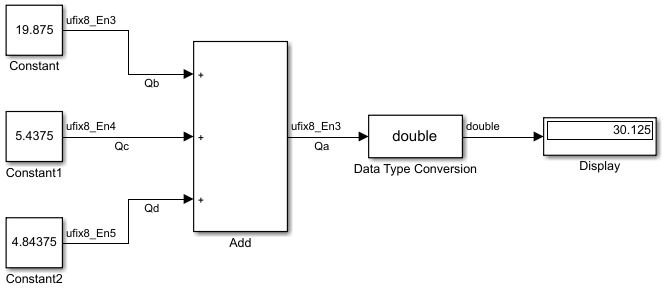## The Summation Process

Addition is the most common arithmetic operation a processor performs. When two n-bit numbers are added together, it is always possible to produce a result with n + 1 nonzero digits due to a carry from the leftmost digit.

Suppose you want to sum three numbers. Each of these numbers is represented by an 8-bit word, and each has a different binary-point-only scaling. Additionally, the output is restricted to an 8-bit word with binary-point-only scaling of 2-3.

The summation is shown in the following model for the input values 19.875, 5.4375, and 4.84375.The sum follows these steps:

1. Because the biases are matched, the initial value of Qa is trivial:

`${Q}_{a}=00000.000.$`
2. The first number to be summed (19.875) has a fractional slope that matches the output fractional slope. Furthermore, the binary points and storage types are identical, so the conversion is trivial:

`$\begin{array}{l}{Q}_{b}=10011.111,\\ {Q}_{Temp}={Q}_{b}.\end{array}$`
3. The summation operation is performed:

`${Q}_{a}={Q}_{a}+{Q}_{Temp}=10011.111.$`
4. The second number to be summed (5.4375) has a fractional slope that matches the output fractional slope, so a slope adjustment is not needed. The storage data types also match, but the difference in binary points requires that both the bits and the binary point be shifted one place to the right:

`$\begin{array}{l}{Q}_{c}=0101.0111,\\ {Q}_{Temp}=convert\left({Q}_{c}\right)\\ {Q}_{Temp}=00101.011.\end{array}$`

Note that a loss in precision of one bit occurs, with the resulting value of QTemp determined by the rounding mode. For this example, round-to-floor is used. Overflow cannot occur in this case because the bits and binary point are both shifted to the right.

5. The summation operation is performed:

Note that overflow did not occur, but it is possible for this operation.

6. The third number to be summed (4.84375) has a fractional slope that matches the output fractional slope, so a slope adjustment is not needed. The storage data types also match, but the difference in binary points requires that both the bits and the binary point be shifted two places to the right:

`$\begin{array}{l}{Q}_{d}=100.11011,\\ {Q}_{Temp}=convert\left({Q}_{d}\right)\\ {Q}_{Temp}=00100.110.\end{array}$`

Note that a loss in precision of two bit occurs, with the resulting value of QTemp determined by the rounding mode. For this example, round-to-floor is used. Overflow cannot occur in this case because the bits and binary point are both shifted to the right.

7. The summation operation is performed:

Note that overflow did not occur, but it is possible for this operation.

As shown here, the result of step 7 differs from the ideal sum:

Blocks that perform addition and subtraction include the Add, Gain, and Discrete FIR Filter blocks.

## SupportGet trial now